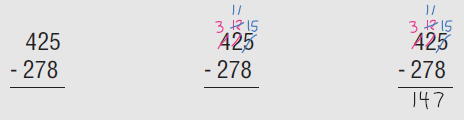# Place Value, Rounding, Addition, and Subtraction

## Objective

Fluently subtract multi-digit whole numbers using the standard algorithm involving up to two decompositions. Solve one-step word problems involving subtraction.

## Materials and Resources

• Base ten blocks  — Optional

## Common Core Standards

### Core Standards

?

• 4.NBT.B.4 — Fluently add and subtract multi-digit whole numbers using the standard algorithm.

?

• 3.NBT.A.2

## Criteria for Success

?

1. Subtract multi-digit numbers with up to two decompositions.
2. Solve one-step word problems involving subtraction with up to one decomposition, using a letter to represent the unknown (MP.4).

## Tips for Teachers

?

• As noted previously, when discussing how to line up numbers in order to add or subtract them vertically, emphasize that units need to be lined up because one can only add or subtract like units (ones with ones and tens with tens), as opposed to saying that numbers need to be lined up from right to left. This is an important distinction since lining numbers up from right to left no longer works when students begin working with decimals (e.g., adding 6.4 and 2.08 would result in an incorrect sum if lined up from right to left).
• It is important not to say things like “you cannot take a bigger number from a smaller number” when discussing subtraction, since this is in fact possible to do, it would just result in a negative number, which students won’t see until Grade 7 (7.NS.1). Instead, you can say things like “there are not enough ones/tens/etc., to subtract.”
• When subtraction requires decomposition, doing all necessary decomposition before subtracting will help to avoid errors. Take 425 – 278, for example. “The total 425 does not have enough tens or ones to subtract the 7 tens or 8 ones in 278. Therefore one hundred is decomposed to make ten tens and one ten is decomposed to make ten ones. These decompositions can be done and written in either order; starting from the left is shown because many students prefer to operate in that order. In the middle step, one hundred has been decomposed (making 3 hundreds, 11 tens, 15 ones) so that the 2 hundreds 7 tens and 8 ones in 278 can be subtracted. These subtractions of like units can also be done in any order. When students alternate decomposing and subtracting like units, they may forget to decompose entirely or in a given column after they have just subtracted (e.g., after subtracting 8 from 15 to get 7, they move left to the tens column and see a 1 on the top and a 7 on the bottom and write 6 because they are in subtraction mode, having just subtracted the ones)” (NBT Progression, p. 10). Thus, decomposing first both allows students to decompose in any order as well as avoid common errors. This process would look like this:• Students have solved one-step word problems involving subtraction in previous grade levels (1.OA.1, 2.OA.1, 3.OA.8), so the intention here is to have students solve contextual problems that involve computations expected of this grade level (4.NBT.4) as well as prepare students to solve multi-step word problems involving addition and subtraction later in the unit, and eventually multiplication (Unit 3) and division (Unit 4) (4.OA.3).

#### Fishtank Plus

• Problem Set
• Student Handout Editor
• Vocabulary Package

?

### Problem 1

Sets of utensils for lunch come in cartons of ten. Those cartons of ten come in boxes of ten. Those boxes of ten come in packages of ten.

Ms. Ruizdeporras has 4 packages, 2 boxes, 5 cartons, and 9 individual sets. After one week, she has 2 packages, 1 box, 7 cartons, and 1 individual sets left. How many sets of utensils did Ms. Ruizdeporras use in one week?

#### References

EngageNY Mathematics Grade 4 Mathematics > Module 1 > Topic E > Lesson 13Concept Development

Grade 4 Mathematics > Module 1 > Topic E > Lesson 13 of the New York State Common Core Mathematics Curriculum from EngageNY and Great Minds. © 2015 Great Minds. Licensed by EngageNY of the New York State Education Department under the CC BY-NC-SA 3.0 US license. Accessed Dec. 2, 2016, 5:15 p.m..

Modified by The Match Foundation, Inc.

### Problem 2

Estimate. Then solve.

1.    __________ = 22,397 – 3,745
2.    260,290 = 45,720 + __________

### Problem 3

The paper mill produced 73,458 boxes of paper this month. Last month, it produced 65,606 boxes of paper. How many more boxes of paper did it produce this month compared to last month?

## Discussion of Problem Set

?

• Compare #1(a) and (f). Does having a larger whole in 1(a) give an answer greater than or less than 1(f)?
• What pattern did you notice between #1(d) and (e)?
• Look at #3. What mistake did Danny make? What should his answer have been?
• What values did you come up with for #7(a)? (b)? (c)? (d)?
• When do we need to unbundle to subtract?
• What are the advantages and disadvantages to modeling subtraction using a place value chart? With the standard algorithm?
• Why must the units line up when subtracting? How might our answer change if the digits were not aligned?

?

Solve. Show or explain your work.

 a.       8,512       -   2,501 b.   18,042 - 4,122 = ${\square}$ c.      19,850      -   15,761

#### References

EngageNY Mathematics Grade 4 Mathematics > Module 1 > Topic E > Lesson 13Exit Ticket

Grade 4 Mathematics > Module 1 > Topic E > Lesson 13 of the New York State Common Core Mathematics Curriculum from EngageNY and Great Minds. © 2015 Great Minds. Licensed by EngageNY of the New York State Education Department under the CC BY-NC-SA 3.0 US license. Accessed Dec. 2, 2016, 5:15 p.m..

Modified by The Match Foundation, Inc.

?